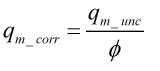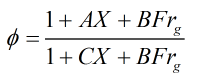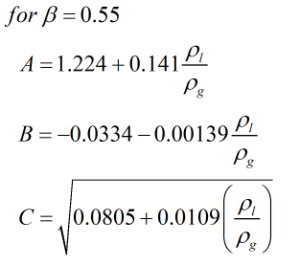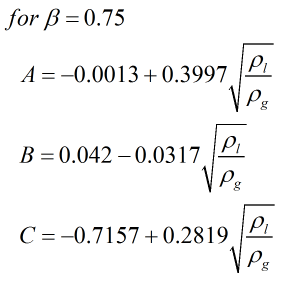# C283 – ISO 5167/McCrometer Wet Gas V-Cone Flow Calculation (Steven)

## Description

This calculates the flow rate of a wet gas through a v-cone meter.

ISO 5167: Measurement of fluid flow by means of pressure differential devices inserted in circular cross-section conduits running full

ISO TR9464: Guidelines for the use of ISO 5167

24509-54 Flow Calculations for the V-Cone and Wafer-Cone Flow meters – McCrometer

24517-16 V-Cone Flow Meter Technical Brief – McCrometer

Wet Gas Metering with V-Cone Meters – R Steven, RJW Peters, D Hodges, D Stewart

Kelton calculation reference C283

FLOCALC calculation reference F099

## Options

### Discharge coefficient

• User entered
• Variable with Reynold’s number

This option gives the user the choice of calculating discharge coefficient against Reynold’s number using linear interpolation or entering a key pad value.

### Standard version

• 2000
• 2005

This option group allows selection of the year of McCrometer technical publications with regard to their v-cone meters.

### Expansibility factor

• User entered
• Calculated

This option gives the choice for expansibility factor to be entered by the user or calculated.

### Beta Ratio

• 0.55
• 0.75

This option is used so that the appropriate equations are used which are dependant on beta ratio.

### Determine Lockhart-Martinelli

• Enter total mass flow rate
• Enter liquid mass flow rate
• Enter gas mass fraction

This option is used to select how the Lockhart-Matinelli parameter is to be calculated based on the data available to the user.

## Calculation

### Corrected Mass Flow Rate

The corrected mass flow rate is calculated by an iterative procedure using the flowing formulae:Where qm_unc = uncorrected gas mass flow rate Φ = wet gas correction factor

The calculations for uncorrected flow rate can be found in C272.

### Wet Gas correction

The wet gas correction is calculated by:Where X = Lockhart-Martinelli parameter Frg = Gas Froude number

And Constants A, B and C are determined by:Where ρg = Gas density ρl = Liquid density

### Lockhart Martinelli Parameter

The Lockhart-Martinelli parameter is calculated depending on the option selected from either:orWhere χ = Gas mass fraction ρg = Gas density ρl = Liquid density qm_gas = gas mass flow rate qm_liq = liquid mass flow rate

### Froude number

The gas densiometric Froude number it calculated by:Where v = Superficial gas velocity D = Corrected pipe diameter g = Gravitational acceleration

Back